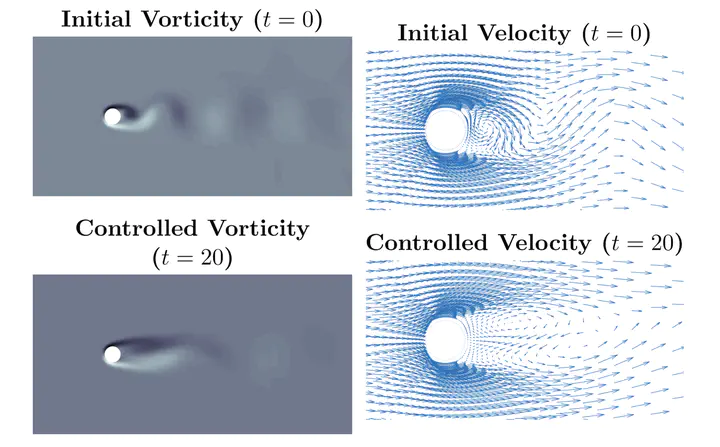# Randomized sketching algorithms for low-memory dynamic optimizationOptimal control of fow past a cylinder.

### Abstract

This paper develops a novel limited-memory method to solve dynamic optimization problems. The memory requirements for such problems often present a major obstacle, particularly for problems with PDE constraints such as optimal fow control, full waveform inversion, and optical tomography. In these problems, PDE constraints uniquely determine the state of a physical system for a given control; the goal is to fnd the value of the control that minimizes an objective. While the control is often low dimensional, the state is typically more expensive to store. This paper suggests using randomized matrix approximation to compress the state as it is generated and shows how to use the compressed state to reliably solve the original dynamic optimization problem. Concretely, the compressed state is used to compute approximate gradients and to apply the Hessian to vectors. The approximation error in these quantities is controlled by the target rank of the sketch. This approximate frst- and second-order information can readily be used in any optimization algorithm. As an example, we develop a sketched trust-region method that adaptively chooses the target rank using a posteriori error information and provably converges to a stationary point of the original problem. Numerical experiments with the sketched trust-region method show promising performance on challenging problems such as the optimal control of an advection-reaction-difusion equation and the optimal control of fuid fow past a cylinder.

Type
Publication
In SIAM Journal on Optimization